# Difference between revisions of "Torsion is tensorial"

This article gives the statement, and possibly proof, that a map constructed in a certain way is tensorial
View other such statements

## Statement

### Symbolic statement

Let$M$ be a differential manifold and$\nabla$ be a linear connection on$M$ (viz.,$\nabla$ is a connection on the tangent bundle$TM$ of$M$).

Consider the torsion of$\nabla$, namely: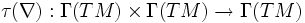$\tau(\nabla): \Gamma(TM) \times \Gamma(TM) \to \Gamma(TM)$

given by: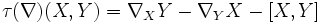$\tau(\nabla)(X,Y) = \nabla_X Y - \nabla_Y X - [X,Y]$

Then,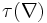$\tau(\nabla)$ is a tensorial map in both coordinates.

## Facts used

• Leibniz rule for derivations: This states that for a vector field$X$ and functions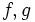$f,g$, we have: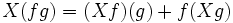$\! X(fg) = (Xf)(g) + f(Xg)$

• Corollary of Leibniz rule for Lie bracket: This states that for a function$f$ and vector fields$X,Y$: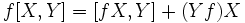$\! f[X,Y] = [fX,Y] + (Yf)X$$\! f[X,Y] = [X,fY] - (Xf)Y$

• The Leibniz rule axiom that's part of the definition of a connection, namely: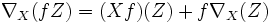$\! \nabla_X(fZ) = (Xf)(Z) + f\nabla_X(Z)$

## Proof

### Tensoriality in the first coordinate

We'll use the fact that tensoriality is equivalent to$C^\infty$-linearity.

To prove: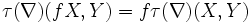$\tau(\nabla)(fX,Y) = f\tau(\nabla)(X,Y)$

Proof: We prove this by expanding everything out on the left side:$\tau(\nabla)(fX,Y) = \nabla_{fX}(Y) - \nabla_Y(fX) - [fX,Y] = f \nabla_X Y - f \nabla_Y X - (Yf)(X) - [fX,Y]$

To prove the equality with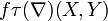$f \tau(\nabla)(X,Y)$, we observe that it reduces to showing:$\! (Yf)(X) = f[X,Y] - [fX,Y]$

which is exactly what the corollary of Leibniz rule above states.

### Tensoriality in the second coordinate

The proof is analogous to that for the first coordinate.

To prove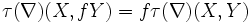$\tau(\nabla)(X,fY) = f \tau(\nabla)(X,Y)$

Proof: We prove this by expanding everything out on the left side: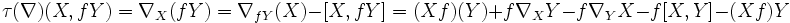$\tau(\nabla)(X,fY) = \nabla_X(fY) = \nabla_{fY}(X) - [X,fY] = (Xf)(Y) + f \nabla_XY - f\nabla_YX - f[X,Y] - (Xf)Y$

(the last step uses the corollary of Leibniz rule).

Canceling terms, yields the required result.